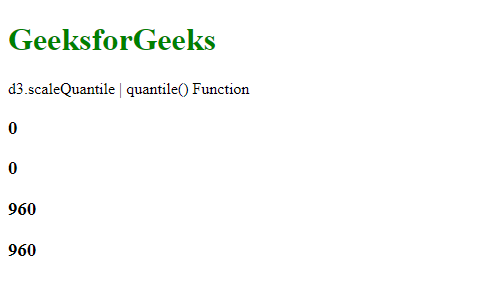# D3.js scaleQuantile quantile() Function

The quantile() function of d3.scaleQuantile() in D3.js is used to return a value from the range corresponding to the given input value. This input value lies in the specified domain.

Syntax:

`quantile( value )`

Parameters: This function accepts a single parameter as given above and described below:

• value: It takes a numeric value that lies in the domain.

Return Values: This function return a value corresponding to the specified range.

The below program illustrates the quantile() function in D3.js:

Example:

## HTML

 ` ` `<``html``> ` ` `  `<``head``> ` `    ``<``script` `src``=``"https://d3js.org/d3.v4.min.js"``> ` `    `` ` `    ``<``script` `src``=``"https://d3js.org/d3-color.v1.min.js"``> ` `    `` ` `    ``<``script` `src``= ` `    ``"https://d3js.org/d3-interpolate.v1.min.js"``> ` `    `` ` `    ``<``script` `src``= ` `    ``"https://d3js.org/d3-scale-chromatic.v1.min.js"``> ` `    `` ` ` ` ` `  `<``body``> ` `    ``<``h1` `style``=``"color: green"``>GeeksforGeeks ` ` `  `    ``<``p``>d3.scaleQuantile | quantile() Function ` ` `  `    ``<``script``> ` `        ``var quantile = d3.scaleQuantile() ` ` `  `            ``// Setting domain and range ` `            ``// for the scale ` `            ``.domain([1, 10]) ` `            ``.range([0, 960]); ` ` `  `        ``// Printing the output using the ` `        ``// quantile() function ` `        ``document.write( ` `            ``"<``h3``>" + quantile(1) + ""); ` `        ``document.write( ` `            ``"<``h3``>" + quantile(5) + ""); ` `        ``document.write( ` `            ``"<``h3``>" + quantile(9) + ""); ` `        ``document.write( ` `            ``"<``h3``>" + quantile(10) + ""); ` `    `` ` ` ` ` `  ` `

Output:Whether you're preparing for your first job interview or aiming to upskill in this ever-evolving tech landscape, GeeksforGeeks Courses are your key to success. We provide top-quality content at affordable prices, all geared towards accelerating your growth in a time-bound manner. Join the millions we've already empowered, and we're here to do the same for you. Don't miss out - check it out now!

Previous
Next